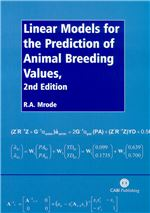Cookies on CAB eBooks

Like most websites we use cookies. This is to ensure that we give you the best experience possible.

Continuing to use www.cabi.org  means you agree to our use of cookies. If you would like to, you can learn more about the cookies we use.

CAB eBooks

Ebooks on agriculture and the applied life sciences from CAB International

CABI Book Info

Linear models for the prediction of animal breeding values.Description

This second edition presents the use of linear models, particularly Best Linear Unbiased Prediction (BLUP), for the genetic evaluation of domestic livestock. The 13 chapters discuss genetic evaluation with different sources of records, genetic covariance between relatives, BLUP of breeding value using univariate models with one random effect, BLUP of breeding value using models with random environmental effects, BLUP of breeding value using multivariate models, maternal trait models, analysis of longitudinal data, the use of genetic markers in predicting breeding values, non-additive animal models, analysis of ordered categorical traits, estimation of genetic parameters, the application of Gibbs sampling in variance component estimation and prediction of breeding value and solving linear equations. Appendices with additional information on introductory matrix algebra, fast algorithms for calculating inbreeding, methods for obtaining approximate reliability for genetic evaluations, procedure for computing de-regressed breeding values, calculating the φ matrix of legendre polynomials at different ages or time periods and computing the covariance matrix of additive genetic effect of marked quantitative trait loci (MQTL) are also included. This book will be of interest to advanced students and practitioners of animal breeding and genetics.

Metrics

Book Chapters

 Chapter: 1 (Page no: 1) Genetic evaluation with different sources of records. Author(s): Mrode, R. A. Chapter: 2 (Page no: 25) Genetic covariance between relatives. Author(s): Mrode, M. R. Chapter: 3 (Page no: 39) Best linear unbiased prediction of breeding value: univariate models with one random effect. Author(s): Mrode, M. R. Chapter: 4 (Page no: 71) Best linear unbiased prediction of breeding value: models with random environmental effects. Author(s): Mrode, M. R. Chapter: 5 (Page no: 83) Best linear unbiased prediction of breeding value: multivariate models. Author(s): Mrode, M. R. Chapter: 6 (Page no: 121) Maternal trait models: animal and reduced animal models. Author(s): Mrode, R. A. Chapter: 7 (Page no: 135) Analysis of longitudinal data. Author(s): Mrode, M. R. Chapter: 8 (Page no: 163) Use of genetic markers in prediction of breeding values. Author(s): Mrode, M. R. Chapter: 9 (Page no: 193) Non-additive animal models. Author(s): Mrode, M. R. Chapter: 10 (Page no: 211) Analysis of ordered categorical traits. Author(s): Mrode, M. R. Chapter: 11 (Page no: 235) Estimation of genetic parameters. Author(s): Thompson, R. Chapter: 12 (Page no: 247) Application of Gibbs sampling in variance component estimation and prediction of breeding value. Author(s): Mrode, M. R. Chapter: 13 (Page no: 259) Solving linear equations. Author(s): Mrode, M. R. Chapter: 14 (Page no: 289) Appendix A: introductory matrix algebra. Author(s): Mrode, M. R. Chapter: 15 (Page no: 297) Appendix B: fast algorithms for calculating inbreeding based on the L matrix. Author(s): Mrode, M. R. Chapter: 16 (Page no: 303) Appendix C. Author(s): Mrode, M. R. Chapter: 17 (Page no: 307) Appendix D: methods for obtaining approximate reliability for genetic evaluations. Author(s): Mrode, M. R. Chapter: 18 (Page no: 311) Appendix E. Author(s): Mrode, M. R. Chapter: 19 (Page no: 317) Appendix F: procedure for computing deregressed breeding values. Author(s): Mrode, M. R. Chapter: 20 (Page no: 321) Appendix G: calculating Φ, a matrix of legendre polynomials evaluated at different ages or time periods. Author(s): Mrode, M. R. Chapter: 21 (Page no: 325) Appendix H: computing the covariance matrix of additive genetic effect of marked QTL (MQTL) when paternal or maternal origin of marker alleles cannot be determined and marker information is incomplete. Author(s): Mrode, M. R.

Book details

• Author Affiliation
• Scottish Agricultural College, Sir Stephen Watson Building, Bush Estate, Penicuik, Midlothian EH26 0PH, UK.
• Year of Publication
• 2005
• ISBN
• 0851990002
• Record Number
• 20053196805